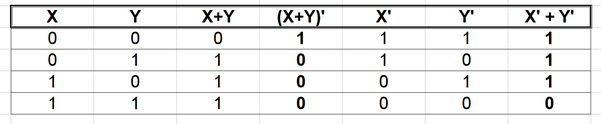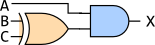# Quiz 3 Solutions

Wed Feb 25

You have up to 20 minutes. You may not use text book or notes.

1. +8 For any Boolean values $$X$$ and $$Y$$, can $$(X+Y)'$$ be rewritten as $$X' + Y'$$?
yes / no

Justify your answer by creating a truth table to show the results of the two expressions for all possible values of $$X$$ and $$Y$$.

The two expressions are not the same, because they have different values in the truth table (see the two columns in bold).2. +6 In algebra, an operator is commutative if the order of its operands can be reversed. For example, standard addition is commutative because $$A+B = B+A$$ for all numbers $$A$$ and $$B$$. Division is not commutative. For example, $$\frac45 \neq \frac54$$ or in decimal notation, $$0.8 \neq 1.25$$.

Which of the Boolean operators (AND, OR, XOR) are commutative?

All of those operators are commutative.

3. +6 Write the Boolean expression implemented by the following circuit diagram.Diagram for question 3

$$A \cdot (B \oplus C)$$